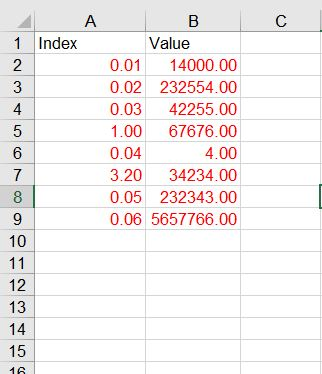# How to filter value using query instead of filter data table

Hi Guys!!

Here I have to filter the values based on the “Index” column. i.e…I have to filter the line items that starts with “0.” can we have any query option to filter the line items instead of using filter data table & for each row activities.Help me if u knowHi @mohamedalthaf
Use this linq query to do that

``````1. First read the excel and store in dt1

2. use assign activity assigning dt2 variable with below value which filters the datatable

dt2= dt1.AsEnumerable().Where(Function(row) row("Index").ToString.Trim.StartsWith("0")).CopyToDataTable

``````

Thanks & Regards,
Nived N

1 Like

Hi, you can use linq for this

``````yourDt = yourDt.AsEnumerable.Where(Function(x) x.Item("Index").ToString.StartsWith("0")).CopyToDataTable
``````

But, before make assignment, make sure that the DT has least one row with your condition or it will be throw a exception in CopyDataTable method.

put in if condition

``````yourDt.AsEnumerable.Where(Function(x) x.Item("Index").ToString.StartsWith("0")).Any
``````

Or you can directly put in assign using if

``````your dt = if(yourDt.AsEnumerable.Where(Function(x) x.Item("Index").ToString.StartsWith("0")).Any, yourDt.AsEnumerable.Where(Function(x) x.Item("Index").ToString.StartsWith("0")).CopyToDataTable, Nothing)
``````

And check later if yourDt is not Nothing

2 Likes

@rikulsilva Thank You

is it possible add multiple filter in this query. for ex: now i am filtering the line items that starts with “0.” I also have to filter the line items starts with “1.0”

How can I do it?

Yes, it is.

``````yourDt = yourDt.AsEnumerable.Where(Function(x) x.Item("Index").ToString.StartsWith("0") or x.Item("Index").ToString.StartsWith("1.0")).CopyToDataTable
``````
1 Like

dtResult =

``````From d in YourDataTabeVar.AsEnumerable
Let si = d.Item("Index").ToString.Trim
Where {"0","1.0"}.Any(Function (x) si.StartsWith(x))
Select r = d).CopyToDataTable
``````
3 Likes

hello try below query:

dt_sample.Select(“[Index]<=1”).CopyToDataTable

2 Likes

This topic was automatically closed 3 days after the last reply. New replies are no longer allowed.